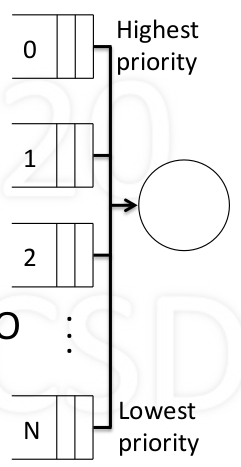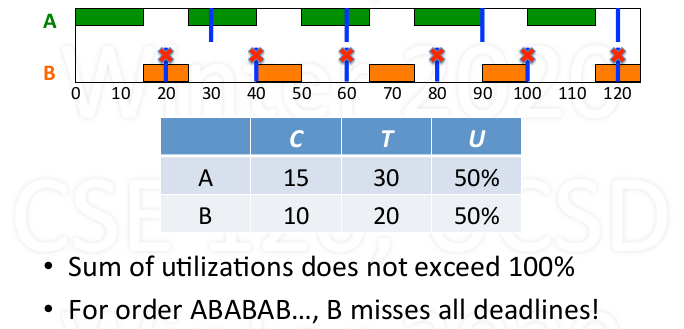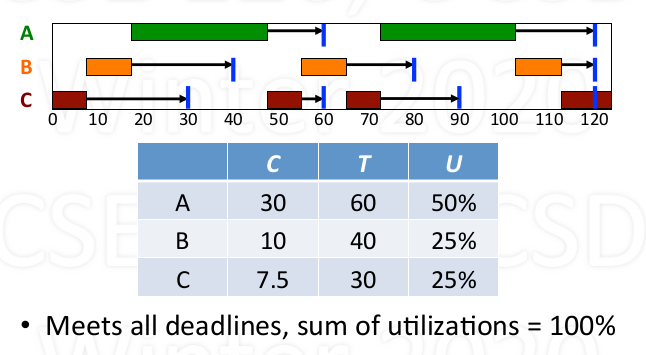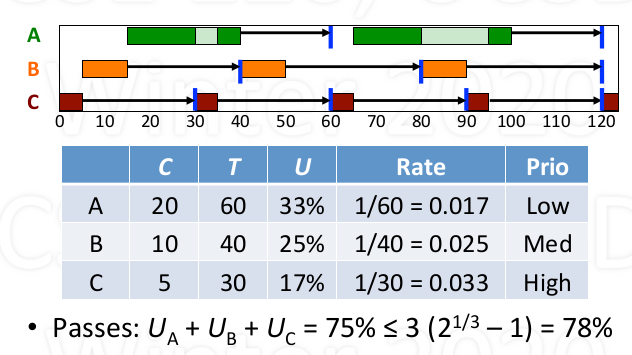0%

## Basic problem and goal

1. Problem introduction

• Given multiple processes and one CPU, which process gets CPU and when?
• How much CPU time does each process get?
• Possible approaches:
• Let one process keep CPU till done then switch to another
• Each process uses CPU a bit and passed it on
• Ech process gets proportinal to what they pay (demand)
• Which policy(ies) is the best?
• Depends on the goals of the system
• Personal computer
• Large time-shared computer
• computer controlling a nuclear power plant…
• Even one system might have multiple(and somtimes conflicting) goals
2. Some parameters needed in scheduling

• Arrival time: time that process is created
• Service time: CPU time needed to complete (most time unknown to the kernel)
• Turnaround time: from arrival to departure (actually time needed to finish the process, including running time and waiting time)
• Try to minimizes average turnaround time

## Different scheduling policies

### Let one process run till done (non-preemptive)

1. Consider the service time for each process (suppose each process arrived at the same time)

• Longest first vs shortest first: Let the longest/shortest processes among all the process created and not yet exit to run till done and then decide the next
• Proven: Shortest first is optimal
• However, the service time is unknown to the kernel in most of the time
2. Consider the arrival time

• First come first serve(FCFS) vs Last come first serve(LCFS): Allocate the CPU to process arrived earliest or latest.
• First come first serve(FCFS)
• non-preemptive, simple, no stavation
• poor for short process arrived late
• Last come first serve(LCFS)
• simple
• starvation, poor for short process arrived early
3. Shortest process next (SPN)

• when one process finish, select the process with shortest service time
• Proven: optimal for non-preemptive policies
• may cause starvation (when short process keep arriving, long process get no chance to run)
• However, the service time is unknown to the kernel in most of the time

### Select process when each quantum end (preemptive)

1. Round Robin (RR)

• Time-slice: each process gets quantum in turn
• Preemptive, simple, no starvation
• Each process waits at most (n - 1) x quantum (supposed n is fixed)
2. Shortest remaing time (SRT)

• At the end of each quantum, select process with shortest remaining time
• Proven: optimal for preemptive policies
• may cause starvation (same case as SPN)
• Assumes service times are known (which is difficult)
3. Multi-level feedback queues• Priority queues 0 to N (from high to low)
• new processes enter queue 0 (highest priority)
• Each quantum select from highest priority queue (FIFO within the queue)
• For each process selected, run it for $T = 2^k$ quantums
• if the process used T quantums, move it next loewer queue
• if the process used less than T quantums, back to same queue
• due to yield or higher priority arrival
• Periodically boost (all to the queue 0)
• Features:
• Complex, adaptive, highly responsive
• Favors shorter over longer, possible starvation (higher priority queue run shorter time)
• Example4. Priority scheduling

• Select process with highest priority
• Calculate priority based some external criteria
• E.g., priority = $\frac{1}{CPU_{time used}}$
5. Fair share (Proportional share)

• Assumed ach process requests ome CPU utilization
• Goal: utilization over long run, actual $\approx$ request
• Select process with minimum actual/request ratio, when some processes have same minumum ratio, randomly choose one.
• involving float number calculation in each quantum, maybe over head.
6. Stride shceduling (practical implementation for Fair share)

• For each process x with certain CPU utilization requested, calculate strides: $S_x = \frac{1}{R_x}$
• For each process x maintain pass value $P_x$ (initialized 0)
• In each quantum:
• Select process x with minimum pass value P to run
• Increment pass value with selected process by its stride value: $P_x = P_x + S_x$
• Optimization: use only intergers for $R_x, S_x, P_x$
• Calucalte $S_x = \frac{L}{R_x}$, where L is very large like 1000000.

### Real Time Scheduling

#### Basics

• Reason (correctness) for real-time scheduling

• depends on logical result of computations
• timeing for these result
• Type of real-time systems

• hard vs. soft real-time
• Periodic vs. aperiodic

#### Type of processes

• Periodic Process (Tasks)

• A periodic process has a fixed frequency at which it needs to run.
• Before each deadline it must run for a certain CPU time
• For each process with a period, we have C (CPU burst needed), T (period), U (C/T, utilization)
• Example• Aperiodic Process

• Aperiodic processes have no fixed, cyclical, frequency between events.
• For this type of process, real-time scheduling is not necessary

#### Different real-time Scheduling

1. Earliest Deadline First (EDF)

• schedule process with earliest deadline
• if a earlier deadline process appears, preempt
• Pros:
• works for periodic and aperiodic processes
• Achieve 100% utilization (igoniring overhead)
• Cons:
• Expensive: requires ordering by deadline frequently
• Example:2. Rate Monotonoic Scheduling (RMS)

• If periodic processes exist, priorityize based on rates
• At start of period, select highest priority
• Preempty if necessary
• When burst done, wait till next period
• Deadline met require: $U_1 + … + U_n \leq n (2 ^ {1/n} - 1)$
• Example:3. More on RMS

• RMS is simple and efficient (static priority)
• RMS is optimal for static priority algorithms
• if RMS can’t schedule, no other static priority can
• RMS is limited in what it guarantees
• Utilization bounded by $n(2^{1/n}-1) > \ln{2}$ ~ 69%
• if bounded exceeded, no guarantess (but may not fail)
• RMS is limited to periodic processes

### Summary

scheduling policy feature
FCFS very simple, non-preemptive
RR simple, preemptive
SPN threoretical, non-preemptive
SRT threoretical, preemptive
MLFQ adaptive, reponsive, complex
Priority external criteria
FS proportional allocation
EDF 100% utilization, high overhead
RMS < 100%, low overhead